# 1.4 Arrays

A data structure is a way to organize data that we wish to process with a computer program. A one-dimensional array (or array) is a data structure that stores a sequence of (references to) objects. We refer to the objects within an array as its elements. The method that we use to refer to elements in an array is numbering and then indexing them. If we have n elements in the sequence, we think of them as being numbered from 0 to n - 1. Then, we can unambiguously specify one of them by referring to the ith element for any integer i in this range.

A two-dimensional array is an array of (references to) one-dimensional arrays. Whereas the elements of a one-dimensional array are indexed by a single integer, the elements of a two-dimensional array are indexed by a pair of integers: the first specifying a row, and the second specifying a column.

## Arrays in Python

The simplest way to create an array in Python is to place comma-separated literals between matching square brackets. For example, the code

 ```SUITS = ['Clubs', 'Diamonds', 'Hearts', 'Spades'] x = [0.30, 0.60, 0.10] y = [0.50, 0.10, 0.40] ```

creates an array `SUITS[]` with four strings, and creates arrays `x[]` and `y[]`, each with three floats.

Given two vectors of the same length, their dot product is the sum of the products of their corresponding components. If we represent the two vectors as one-dimensional arrays `x[]` and `y[]` that are each of length n, their dot product is easy to compute:

 ```total = 0.0 for i in range(n): total += x[i]*y[i] ```

For example, following trace shows the computation of the dot product of two vectors of length 3.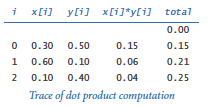It is useful to think of references to the elements in an array as stored contiguously, one after the other, in your computer's memory, as shown in the diagram at the right for the `SUITS[]` array defined above.

### Zero-based indexing.

We always refer to the first element of an array a[] as a, the second as a, and so forth. It might seem more natural to refer to the first element as a, the second element as a, and so forth, but starting the indexing with 0 has some advantages and has emerged as the convention used in most modern programming languages.

### Array length.

You can access the length of an array using Python's built-in `len()` function: `len(a)` is the number of elements in `a[]`. In Python, we can use the `+=` operator to append elements to an array. For example, if `a[]` is the array `[1, 2, 3]`, then the statement `a += ` extends it to `[1, 2, 3, 4]`. More generally, we can make an array of `n` floats, with each element initialized to `0.0`, with the code

 ```a = [] for i in range(n): a += [0.0] ```

### Bounds checking.

You must be careful when programming with arrays. It is your responsibility to use legal indices when accessing an array element.

### Mutability.An object is mutable if its value can change. Arrays are mutable objects because we can change their elements. For example, if we create an array with the code `x = [.30, .60, .10]`, then the assignment statement `x = .99` changes it to the array `[.30, .99, .10]`. An object-level trace of this operation is shown at the right.

The following code reverses the order of the elements in an array a[]:

 ```n = len(a) for i in range(n // 2): temp = a[i] a[i] = a[n-1-i] a[n-1-i] = temp ```

An informal trace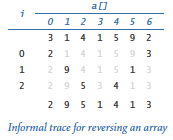of this code for a seven-element array `[3, 1, 4, 1, 5, 9, 2]` is shown at the right.

### Iteration.

The following code iterates over all elements of an array to compute the average of the floats that it contains:

 ```total = 0.0 for i in range(len(a)): total += a[i] average = total / len(a) ```

Python also supports iterating over the elements in an array without referring to the indices explicitly. To do so, put the array name after the `in` keyword in a `for` statement, as follows:

 ```total = 0.0 for v in a: total += v average = total / len(a) ```

### Built-in functions.

Python has several built-in functions that can take arrays as arguments. We have already discussed the `len()` function. As another example, if the elements of `a[]` are numeric, then sum(a) computes their sum, so that we can compute their average with `float(sum(a)) / len(a)` instead of using either of the loops just described. Other useful built-in functions that can take arrays as arguments are `min()` for computing the minimum and `max()` for computing the maximum.

### Writing an array.

You can write an array by passing it as an argument to `stdio.write()` or `stdio.writeln()`. Each object in the array is converted to a string.

## Array Aliases and Copies

Before looking at programs that use arrays, it is worthwhile to examine two fundamental array-processing operations in more detail.

### Aliasing.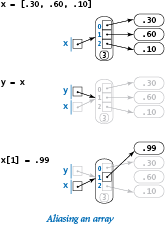If `x[]` and `y[]` are arrays, the statement `x = y` causes `x` and `y` to reference the same array. This result has an effect that is perhaps unexpected, at first, because it is natural to think of `x` and `y` as references to two independent arrays. For example, after the assignment statements

 ```x = [.30, .60, .10] y = x x = .99 ```

`y` is also `.99`, even though the code does not refer directly to `y`. This situation — whenever two variables refer to the same object — is known as aliasing, and is illustrated in the object-level trace at the right.

### Copying and slicing.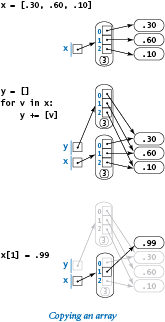So how do we make a copy `y[]` of a given array `x[]`? One answer to this question is to iterate through `x[]` to build `y[]`, as in the following code:

 ```y = [] for v in x: y += [v] ```

This situation is illustrated in the object-level trace at the right.

Copying an array is such a useful operation that Python provides language support for a more general operation known as slicing, The expression `a[i:j]` evaluates to a new array whose elements are `a[i], ..., a[j-1]`. Moreover, the default value for `i` is 0 and the default value for `j` is `len(a)`, so `y = x[:]` is equivalent to the code given earlier.

## System Support for Arrays

Python code for processing arrays can take many forms. We describe each briefly for context.

### Python's built-in `list` data type.

In its most basic form, an array supports four core operations: creation, indexed access, indexed assignment, and iteration. In this booksite, we use Python's built-in `list` data type for arrays because it supports these basic operations. We consider more elaborate operations supported by Python's `list` data type in Chapter 4.

### Python's `numpy` module.

Python's built-in `list` data type can have severe performance problems. For that reason, scientists and engineers often use a Python extension module called `numpy` for processing huge arrays of numbers, because that module uses a lower-level representation that avoids many of the inefficiencies in the standard Python representation. See Appendix: `numpy` for an overview of the `numpy` module.

### Our `stdarray` module.

Earlier we introduced the booksite `stdio` module. Now, we introduce another booksite module: the `stdarray` module. Its primary purpose is to define functions for processing arrays.

A fundamental operation that is found in nearly every array-processing program is to create an array of n elements, each initialized to a given value. As we have seen, you can do this in Python with code like the following:

 ```a = [] for i in range(n): a += [0.0] ```

Such code is so common that Python even has a special shorthand notation for it: the code `a = [0.0]*n` is equivalent to the code just given. Rather than repeat such code throughout the book, we will use code like this:

 ```a = stdarray.create1D(n, 0.0) ```

For consistency, `stdarray` also includes a `create2D()` function, which we will examine later in this section.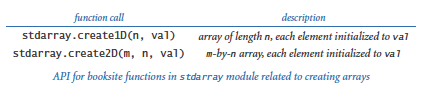## Sample Applications of Arrays

Next, we consider a number of applications that illustrate the utility of arrays and also are interesting in their own right.

### Representing playing cards.

Suppose that we want to compose programs that process playing cards. We might start with the following code:

 ```SUITS = ['Clubs', 'Diamonds', 'Hearts', 'Spades'] RANKS = ['2', '3', '4', '5', '6', '7', '8', '9', '10', 'Jack', 'Queen', 'King', 'Ace'] ```

For example, we might use these two arrays to write a random card name, such as `Queen of Clubs`, as follows:

 ```rank = random.randrange(0, len(RANKS)) suit = random.randrange(0, len(SUITS)) stdio.writeln(RANKS[rank] + ' of ' + SUITS[suit]) ```

A more typical situation is when we compute the values to be stored in an array. For example, we might use the following code to initialize an array of length 52 that represents a deck of playing cards, using the two arrays just defined:

 ```deck = [] for rank in RANKS: for suit in SUITS: card = rank + ' of ' + suit deck += [card] ```

### Exchange.

Frequently, we wish to exchange two elements in an array. Continuing our example with playing cards, the following code exchanges the cards at indices `i` and `j`:

 ```temp = deck[i] deck[i] = deck[j] deck[j] = temp ```

### Shuffle

. The following code shuffles our deck of cards:

 ```n = len(deck) for i in range(n): r = random.randrange(i, n) temp = deck[r] deck[r] = deck[i] deck[i] = temp ```

Proceeding from left to right, we pick a random card from deck[i] through deck[n-1] (each card equally likely) and exchange it with deck[i].

### Sampling without replacement.

In many situations, we want to draw a random sample from a set such that each element in the set appears at most once in the sample. The program sample.py takes command-line arguments `m` and `n` and creates a permutation of size `n` whose first `m` elements constitute a random sample.

### Precomputed values.

Another application of arrays is to save values that you have computed for later use. As an example, suppose that you are composing a program that performs calculations using small values of the harmonic numbers. An efficient approach is to save the values in an array, as follows:

 ```harmonic = stdarray.create1D(n+1, 0.0) for i in range(1, n+1): harmonic[i] = harmonic[i-1] + 1.0/i ```

Note that we waste one slot in the array (element 0) to make harmonic correspond to the first harmonic number 1.0 and harmonic[i] correspond to the ith harmonic number. This method is not effective if we need values for huge n, but it is very effective if we need values for small n many different times.

### Simplifying repetitive code.

As an example of another simple application of arrays, consider the following code fragment, which writes the name of a month given its number (1 for January, 2 for February, and so forth):

 ```if m == 1: stdio.writeln('Jan') elif m == 2: stdio.writeln('Feb') elif m == 3: stdio.writeln('Mar') elif m == 4: stdio.writeln('Apr') ... elif m == 11: stdio.writeln('Nov') elif m == 12: stdio.writeln('Dec') ```

A more compact alternative is to use an array of strings holding the month names:

 ```MONTHS = ['', 'Jan', 'Feb', 'Mar', 'Apr', 'May', 'Jun', 'Jul', 'Aug', 'Sep', 'Oct', 'Nov', 'Dec'] ... stdio.writeln(MONTHS[m]) ```

This technique would be especially useful if you needed to access the name of a month by its number in several different places in your program. Note that we intentionally waste one slot in the array (element 0) to make `MONTHS` correspond to January, as required.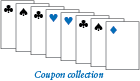### Coupon collector.

Suppose that you have a deck of cards and you pick cards at random (with replacement) one by one. How many cards do you need to turn up before you have seen one of each suit? That is an example of the famous coupon collector problem. In general, suppose that a trading card company issues trading cards with n different possible cards: how many do you have to collect before you have all n possibilities, assuming that each possibility is equally likely for each card that you collect? The program couponcollector.py is an example program that simulates this process. See the textbook for details.

### Sieve of Eratosthenes.

The prime counting function π(n) is the number of primes less than or equal to n. For example π(17) = 7 since the first seven primes are 2, 3, 5, 7, 11, 13, and 17. Program primesieve.py takes a command line integer n and computes π(n) using the Sieve of Eratosthenes. See the textbook for details.

## Two-Dimensional Arrays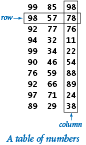In many applications, a convenient way to store information is to use a table of numbers organized in a rectangular table and refer to rows and columns in the table. The mathematical abstraction corresponding to such tables is a matrix; the corresponding data structure is a two-dimensional array.

### Initialization.

The simplest way to create a two-dimensional array is to place comma-separated one-dimensional arrays between matching square brackets. For example, this matrix of integers having two rows and three columns

 ```18 19 20 21 22 23 ```

could be represented in Python using this array of arrays:

 ```a = [[18, 19, 20], [21, 22, 23]] ```

We call such an array a 2-by-3 array. More generally, Python represents an m-by-n array as an array that contains m objects, each of which is an array that contains n objects. For example, this Python code creates an m-by-n array `a[][]` of floats, with all elements initialized to 0.0:

 ```a = [] for i in range(m): row = [0.0] * n a += [row] ```

As for one-dimensional arrays, we use the self-descriptive alternative `stdarray.create2D(m, n, 0.0)` from our booksite module `stdarray` throughout this booksite.

### Indexing.

When `a[][]` is a two-dimensional array, the syntax `a[i]` denotes a reference to its `i`th row. The syntax `a[i][j]` refers to the object at row `i` and column `j`. To access each of the elements in a two-dimensional array, we use two nested `for` loops. For example, this code writes each object of the m-by-n array `a[][]`, one row per line.

 ```for i in range(m): for j in range(n): stdio.write(a[i][j]) stdio.write(' ') stdio.writeln() ```

This code achieves the same effect without using indices:

 ```for row in a: for v in row: stdio.write(v) stdio.write(' ') stdio.writeln() ```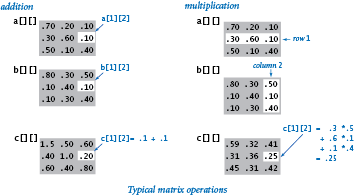### Matrix operations.

Typical applications in science and engineering involve representing matrices as two-dimensional arrays and then implementing various mathematical operations with matrix operands. For example, we can add two n-by-n matrices `a[][]` and `b[][]` as follows:
 ```c = stdarray.create2D(n, n, 0.0) for i in range(n): for j in range(n): c[i][j] = a[i][j] + b[i][j] ```

Similarly, we can multiply two matrices. Each element `c[i][j]` in the product of `a[][]` and `b[][]` is computed by taking the dot product of row `i` of `a[][]` with column `j` of `b[][]`.

 ```c = stdarray.create2D(n, n, 0.0) for i in range(n): for j in range(n): # Compute the dot product of row i and column j for k in range(n): c[i][j] += a[i][k] * b[k][j] ```

### Ragged arrays.

There is actually no requirement that all rows in a two-dimensional array have the same length. An array with rows of nonuniform length is known as a ragged array. The possibility of ragged arrays creates the need for taking more care in crafting array-processing code. For example, this code writes the contents of a ragged array:

 ```for i in range(len(a)): for j in range(len(a[i])): stdio.write(a[i][j]) stdio.write(' ') stdio.writeln() ```

Note that the equivalent code that does not use indices works equally well with both rectangular and ragged arrays:

 ```for row in a: for v in row: stdio.write(v) stdio.write(' ') stdio.writeln() ```

### Multidimensional arrays.

The same notation extends to allow us to compose code using arrays that have any number of dimensions. Using arrays of arrays of arrays..., we can create three-dimensional arrays, four-dimensional arrays, and so forth, and then refer to an individual element with code like `a[i][j][k]`.

### Example: self-avoiding random walks.

The program selfavoid.py is an application of two-dimensional arrays to chemistry. See the textbook for details.

#### Q & A

Q. Why do Python string and list indices start at 0 instead of 1?

A. That convention originated with machine-language programming, where the address of an array element would be computed by adding the index to the address of the beginning of an array. Starting indices at 1 would entail either a waste of space at the beginning of the array or a waste of time to subtract the 1. Here's Edsger Dijkstra's explanation.

Q. What happens if I use a negative integer to index an array?

A. The answer may surprise you. Given an array `a[]`, you can use the index `-i` as shorthand for `len(a)-i`. For example, you can refer to the last element in the array with `a[-1]` or `a[len(a)-1]` and the first element with `a[-len(a)]` or `a`. Python raises an `IndexError` at run time if you use an index outside of the range `-len(a)` through `len(a)-1`.

Q. Why does the slice `a[i:j]` include `a[i]` but exclude `a[j]`?

A. The notation is consistent with ranges defined with `range()`, which includes the left endpoint but excludes the right endpoint. It leads to some appealing properties: `j-i` is the length of the subarray (assuming no truncation); `a[0:len(a)]` is the entire array; `a[i:i]` is the empty array; and a`[i:j] + a[j:k]` is the subarray `a[i:k]`.

Q. What happens when I compare two arrays `a[]` and `b[]` with `(a == b)`?

A. It depends. For arrays (or multidimensional arrays) of numbers, it works as you might expect: the arrays are equal if each has the same length and the corresponding elements are equal.

Q. What happens when a random walk does not avoid itself?

A. This case is well understood. It is a two-dimensional version of the gambler's ruin problem, as described in Section 1.3.

Q. Which pitfalls should I watch out for when using arrays?

A. Remember that creating an array takes time proportional to the length of the array. You need to be particularly careful about creating arrays within loops.

#### Exercises

1. Compose a program that creates a one-dimensional array `a` containing exactly 1000 integers, and then attempts to access `a`. What happens when you run the program?

2. Given two vectors of length `n` that are represented with one-dimensional arrays, compose a code fragment that computes the Euclidean distance between them (the square root of the sum of the squares of the differences between corresponding elements).

3. Compose a code fragment that reverses the order of a one-dimensional array of floats. Do not create another array to hold the result. Hint: Use the code provided earlier in this web page for exchanging two elements.

Solution:

```n = len(a)
for i in range(n//2):
temp = a[n-i-1]
a[n-i-1] = a[i]
a[i] = temp
```
4. What is wrong with the following code fragment?

 ```a = [] for i in range(10): a[i] = i * i ```

Solution: Initially `a` is the empty array. Subsequently no elements are appended to the array. Thus `a`, `a`, and so forth do not exist. The attempts to use them in an assignment statement will raise an `IndexError` at run time.

5. Compose a code fragment that writes the contents of a two-dimensional array of bools, using `*` to represent `True` and a space to represent `False`. Include row and column numbers.

6. What does the following code fragment write?

 ```a = stdarray.create1D(10, 0) for i in range(10): a[i] = 9 - i for i in range(10): a[i] = a[a[i]] for v in a: stdio.writeln(v) ```
7. What is `a[]` afater executing the following code fragment?

 ```n = 10 a = [0, 1] for i in range(2, n): a += [a[i-1] + a[i-2]] ```
8. Compose a program that takes an integer command-line argument `n` and writes `n` poker hands (five cards each) from a shuffled deck, separated by blank lines.

Solution: See deal.py.

9. Compose code fragments to create a two-dimensional array `b[][]` that is a copy of an existing two-dimensional array `a[][]`, under each of the following assumptions:

1. `a` is square.
2. `a` is rectangular.
3. `a` may be ragged.

Your solution to (b) should work for (a), and your solution to (c) should work for both (b) and (a).

10. Compose a code fragment to write the transposition (rows and columns changed) of a two-dimensional array. For the example, when given this two-dimensional array of integers:

 ```99 85 98 98 57 78 92 77 76 94 32 11 99 34 22 90 46 54 76 59 88 92 66 89 97 71 24 89 29 38 ```

 ```99 98 92 94 99 90 76 92 97 89 85 57 77 32 34 46 59 66 71 29 98 78 76 11 22 54 88 89 24 38 ```
11. Compose a code fragment to transpose a square two-dimensional array `b[][]` in place without creating a second array.

Solution. See transpose.py.

12. Compose a code fragment to create a two-dimensional array `b[][]` that is the transpose of an existing m-by-n array `a[][]`.

13. Compose a program that computes the product of two square matrices of boolean values, using the `or` operation instead of `+` and the `and` operation instead of `*`.

14. Compose a program that accepts an integer n from the command line and creates an n-by-n boolean array `a` such that `a[r][c]` is `True` if `r` and `c` are relatively prime (have no common factors other than 1), and `False` otherwise. Then write the array (see a previous exercise in this section of the booksite) using `*` to represent `True` and a space to represent `False`. Include row and column numbers. Hint: Use sieving.

15. Compose a code fragment to multiply two rectangular matrices of floats that are not necessarily square. Note: For the dot product to be well-defined, the number of columns in the first matrix must be equal to the number of rows in the second matrix. Write an error message if the dimensions do not satisfy this condition.

16. Modify selfavoid.py to calculate and write the average length of the paths as well as the dead-end probability. Keep separate the average lengths of escape paths and dead-end paths.

17. Modify selfavoid.py to calculate and write the average area of the smallest axis-oriented rectangle that encloses the path. Keep separate statistics for escape paths and dead-end paths.

#### Creative Exercises

1. Dice simulation. The following code computes the exact probability distribution for the sum of two dice:

```probabilities = stdarray.create1D(13, 0.0)

for i in range(1, 7):
for j in range(1, 7):
probabilities[i+j] += 1.0

for k in range(2, 13):
probabilities[k] /= 36.0
```

After this code completes, `probabilities[k]` is the probability that the dice sum to `k`. Run experiments to validate this calculation simulating n dice throws, keeping track of the frequencies of occurrence of each value when you compute the sum of two random integers between 1 and 6. How large does n have to be before your empirical results match the exact results to three decimal places?

2. Longest plateau. Given an array of integers, compose a program that finds the length and location of the longest contiguous sequence of equal values where the values of the elements just before and just after this sequence are smaller.

3. Empirical shuffle check. Run computational experiments to check that our shuffling code works as advertised. Compose a program that takes integer command-line arguments m and n, does n shuffles of an array of size m that is initialized with `a[i] = i` before each shuffle, and writes an m-by-m table such that row `i` gives the number of times `i` wound up in position `j` for all `j`. All entries in the array should be close to n/m.

4. Bad shuffling. Suppose that you choose a random integer between 0 and n-1 in our shuffling code instead of one between `i` and `n-1`. Show that the resulting order is not equally likely to be one of the n! possibilities. Run the test of the previous exercise for this version.

Partial solution: When n = 3, all 3! = 6 outcomes are possible, but some are more likely:

 ABC ACB BAC BCA CAB CBA 4/27 5/27 6/27 4/27 5/27 3/27
5. Music shuffling. You set your music player to shuffle mode. It plays each of the n songs before repeating any. Compose a program to estimate the likelihood that you will not hear any sequential pair of songs (that is, song 3 does not follow song 2, song 10 does not follow song 9, and so on).

6. Minima in permutations. Compose a program that takes an integer n from the command line, generates a random permutation, writes the permutation, and writes the number of left-to-right minima in the permutation (the number of times an element is the smallest seen so far). Then compose a program that takes integers m and n from the command line, generates m random permutations of size n, and writes the average number of left-to-right minima in the permutations generated. Extra credit: Formulate a hypothesis about the number of left-to-right minima in a permutation of size n, as a function of n.

7. Inverse permutation. Compose a program that accepts a permutation of the integers 0 to n-1 from n command-line arguments and writes its inverse. (If the permutation is an array `a[]`, its inverse is the array `b[]` such that `a[b[i]]` = `b[a[i]]` = `i`.) Be sure to check that the input is a valid permutation.

Solution: See inversepermutation.py.

8. Hadamard matrix. The n-by-n Hadamard matrix Hn matrix is a boolean matrix with the remarkable property that any two rows differ in exactly n/2 elements. (This property makes it useful for designing error-correcting codes.) H1 is a 1-by-1 matrix with the single element `True`, and for n > 1, H2n is obtained by aligning four copies of Hn in a large square, and then inverting all of the elements in the lower right n-by-n copy, as shown in the following examples (with `T` representing `True` and `F` representing `False`, as usual).

 ```H(1) H(2) H(4) ------------------- T T T T T T T T F T F T F T T F F T F F T ```

Compose a program that takes one command-line argument n and writes Hn. Assume that n is a power of 2.

9. Rumors. Alice is throwing a party with n other guests, including Bob. Bob starts a rumor about Alice by telling it to one of the other guests. A person hearing this rumor for the first time will immediately tell it to one other guest, chosen at random from all the people at the party except Alice and the person from whom they heard it. If a person (including Bob) hears the rumor for a second time, he or she will not propagate it further. Compose a program to estimate the probability that everyone at the party (except Alice) will hear the rumor before it stops propagating. Also calculate an estimate of the expected number of people to hear the rumor.

10. Find a duplicate. Given an array of n elements with each element between 1 and n, compose a code fragment to determine whether there are any duplicates. You do not need to preserve the contents of the given array, but do not use an extra array.

11. Minesweeper. Compose a program that takes three command-line arguments m, n, and p and produces an m-by-n boolean array where each element is occupied with probability p. In the minesweeper game, occupied cells represent bombs and empty cells represent safe cells. Write the array using an asterisk for bombs and a period for safe cells. Then, replace each safe square with the number of neighboring bombs (above, below, left, right, or diagonal) and write the result, as in this example.

 ```* * . . . * * 1 0 0 . . . . . 3 3 2 0 0 . * . . . 1 * 1 0 0 ```

Try to express your code so that you have as few special cases as possible to deal with, by using an (m+2)-by-(n+2) boolean array.

Solution: See minesweeper.py.

12. Self-avoiding walk length. Suppose that there is no limit on the size of the grid. Run experiments to estimate the average walk length.

13. Three-dimensional self-avoiding walks. Run experiments to verify that the dead-end probability is 0 for a three-dimensional self-avoiding walk and to compute the average walk length for various values of n.

14. Random walkers. Suppose that `n` random walkers, starting in the center of an n-by-n grid, move one step at a time, choosing to go left, right, up, or down with equal probability at each step. Compose a program to help formulate and test a hypothesis about the number of steps taken before all cells are touched.

Solution: See randomwalkers.py.

15. Bridge hands. In the game of bridge, four players are dealt hands of 13 cards each. An important statistic is the distribution of the number of cards in each suit in a hand. Which is the most likely, 5-3-3-2, 4-4-3-2, or 4-3-3-3? Compose a program to help you answer this question.

16. Birthday problem. Suppose that people continue to enter an empty room until a pair of people share a birthday. On average, how many people will have to enter before there is a match? Run experiments to estimate the value of this quantity. Assume birthdays to be uniform random integers between 0 and 364.

Solution: See birthday.py and birthdays.py.

17. Coupon collector. Run experiments to validate the classical mathematical result that the expected number of coupons needed to collect n values is about nHn. For example, if you are observing the cards carefully at the blackjack table (and the dealer has enough decks randomly shuffled together), you will wait until about 235 cards are dealt, on average, before seeing every card value.

18. Riffle shuffle. Compose a program to rearrange a deck of n cards using the Gilbert-Shannon-Reeds model of a riffle shuffle. First, generate a random integer r according to a binomial distribution: flip a fair coin n times and let r be the number of heads. Now, divide the deck into two piles: the first r cards and the remaining n - r cards. To complete the shuffle, repeatedly take the top card from one of the two piles and put it on the bottom of a new pile. If there are n1 cards remaining in the first pile and n2 cards remaining in the second pile, choose the next card from the first pile with probability n1 / (n1 + n2) and from the second pile with probability n2 / (n1 + n2). Investigate how many riffle shuffles you need to apply to a deck of 52 cards to produce a (nearly) uniformly shuffled deck.

19. Binomial coefficients. Compose a program that builds and writes a two-dimensional ragged array `a` such that `a[n][k]` contains the probability that you get exactly `k` heads when you toss a fair coin `n` times. Take a command-line argument to specify the maximum value of `n`. These numbers are known as the binomial distribution: if you multiply each element in row k by 2n, you get the binomial coefficients (the coefficients of xk in (x+1)n) arranged in Pascal's triangle. To compute them, start with `a[n] = 0.0` for all `n` and `a = 1.0`, then compute values in successive rows, left to right, with `a[n][k] = (a[n-1][k] + a[n-1][k-1])/2.0`.

 ```Pascal's triangle Binomial distribution 1 1 1 1 1/2 1/2 1 2 1 1/4 1/2 1/4 1 3 3 1 1/8 3/8 3/8 1/8 1 4 6 4 1 1/16 1/4 3/8 1/4 1/16 ```